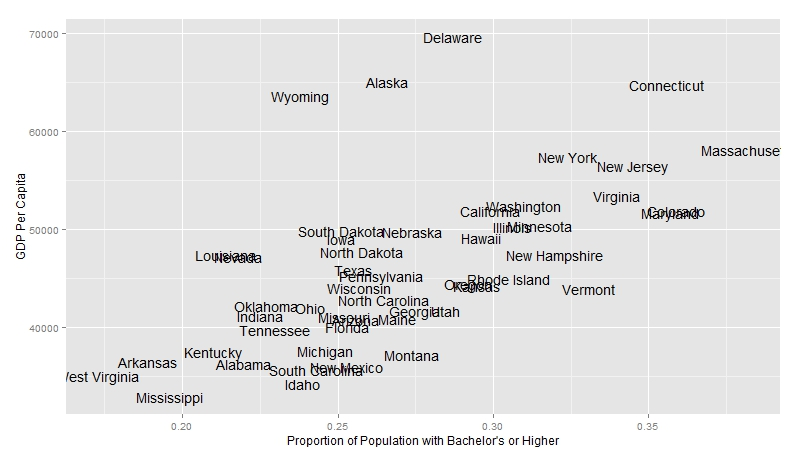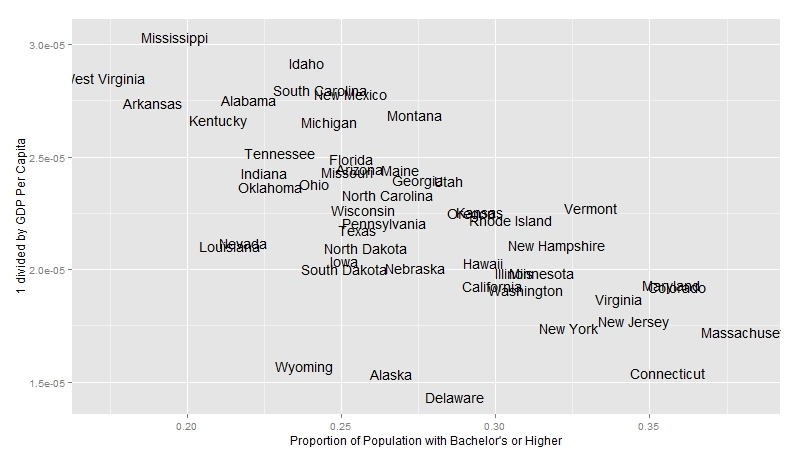Want to share your content on R-bloggers? click here if you have a blog, or here if you don't.

The inspiration for this post came as I was browsing texts and articles about USA’s GDP and I wondered what might have a positive relationship to GDP that would be interesting to graph and explore.

I stumbled upon two datasets: US States by Educational Attainment and US States by GDP. The data looked clean enough so I decided to write up a quick R program to see what I could find.

First I imported the two datasets and merged them into one frame. I then built a linear model using GDP per Capita as the response variable and Percent of the Population with Bachelors are the predictor.

The summary of this simple linear model is featured below:

As we can see, the p-value is very small (small enough for this model to be significant). However the low R-Squared leaves much to be desired. Very little of the variance in the data is accounted for by our statistical model and I question whether it is a good fit or not.

Nevertheless, we can see a pretty interesting graph below:Aha! We can attribute some of our lack of fit to the outliers Wyoming, Alaska, and Delaware. With such a small dataset (50 states in the USA) 3 outliers can definitely have a strong impact on the fit.

I tried a Box-Cox test in R to see whether our fit might be improved if we transformed the response variable. The Box-Cox plot in R suggests that raising the response to the negative first power might be beneficial.

This new model is outlined below:

The p-value of this new model is even lower and the R-Squared value also improved slightly. Still, the model is not perfect and it would be unwise to claim that we have a strong linear relationship between the two.

Graphing this we get:Once again we see the same 3 outliers which definitely has a huge impact on the significance of such a small model. Either way, this model seems to exhibit somewhat of a stronger relationship between the two variables.